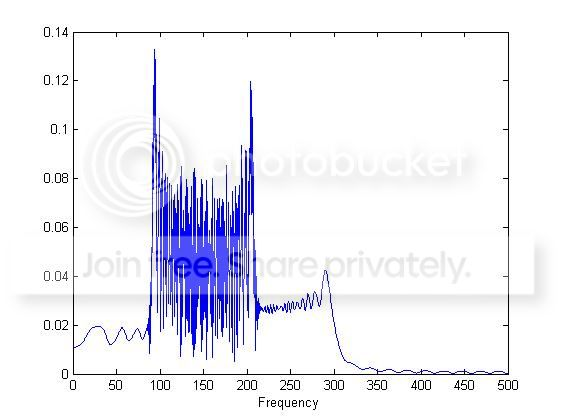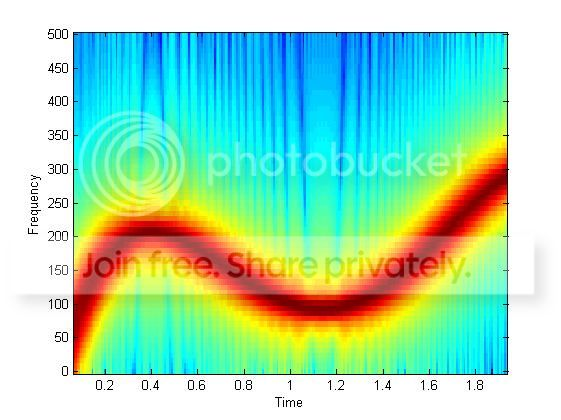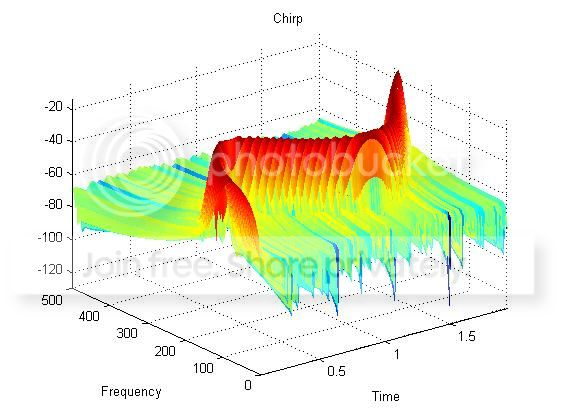# Spectrogram of a chirp and its FFT

This question follows from an answer to this other question: Conceptual question on FFT and chirp signal

I wrote a code starting from the spectrogram to compare it with the FFT result.

% Chirp generation
t = [0 0.5 1.0 1.5 2.0]; % time breakpoints
f = [0 200 100 150 300];  % instantaneous frequency breakpoints
p = polyfit(t,f,4);
dt = 0.001;
t = 0:dt:2;
y = chirp(t,p);
L = length(y);

% FFT
NFFT = 2^nextpow2(L);
Y = fft(y,NFFT)/L;
Fs = 1/dt;
f_plot = Fs/2*linspace(0,1,NFFT/2+1);

% Spectrogram
figure()
specgram(y,128,Fs,128,120); % 2D time-freqency display

[S,F,T,P]=spectrogram(y,128,120,128,1E3);
figure()
surf(T,F,10*log10(P),'edgecolor','none')
axis tight


This is what I obtain.My qyestion is: shouldn't the spectrogram and the FFT have the same amplitude variation? Whereas the spectrogram does not seem to have amplitude variation (the red line is red at the same way everywhere).

Second question, why in the FFT I have high peaks at $100$ and $200 Hz$ only?

• This is just a guess, but it seems like the signal stays at 100 Hz and 200 Hz longer than at other frequencies. For instance, the frequency is very close to 100 Hz in the interval from 1 s to 1.2 s. The FFT reflects this.
– MBaz
Jan 12 '15 at 20:20

FFT calculates spectrum over the whole duration of your signal (integrates energy at each frequency over the time of accumulation) while spectrogram shows frequency variation in time (instantaneous frequency). Divide the whole frequency band of interest in some equal intervals and evaluate how much time signal's frequency falls in each interval. These time periods aren't equal. So by FFT frequencies corresponding to larger time periods gains more power and will be major and vice versa. Now lets look at your spectrogram. We see that it's about 100 and 200 Hz when the most stationarity in frequency exists, there are maximums of the spectrum. Frequencies between 100 and 200 Hz appears 2 times, so we see raising of the spectrum at this span. Low and high frequencies appears only 1 time and frequency at these regions varies faster: the spectrum after FFT decreases.

• Now I see. But one more thing, has the amplitude of the spectrogram something to do with the amplitude of the signal? In my example, since it is a chirp, the amplitude is always 1, but I could have a signal which has a time dependent amplitude also. What I am expecting is that I will see the colors of the spectrogram changing according to the amplitude changes, am I right ?
– Rhei
Jan 13 '15 at 5:53
• Not even mentioning how Frequency Modulation is changing spectra in very non-intuitive way.
– jojek
Jan 13 '15 at 8:50
• Since spectrogram represents signal both in time and frequency the colors will change according to amplitude variation. But it isn't true for FFT. The picture will be different but it won't contain any information about time variation of amplitude since it gives only frequency structure of the signal during its accumulation period
– Serj
Jan 13 '15 at 16:33

If you look at the first derivative of your frequency sweep, you will note that the derivative goes thru zero just below 100 Hz and just above 200 Hz. When the slope of the frequency is near zero, the frequency isn't changing very fast, thus staying longer in those frequency regions, thus having more energy in those frequency regions or bins, thus causing higher peaks in the FFT result.

The spectrogram will show the same variations if you squash it sideways (the triple overlap will sum, the non overlapping frequencies won't).